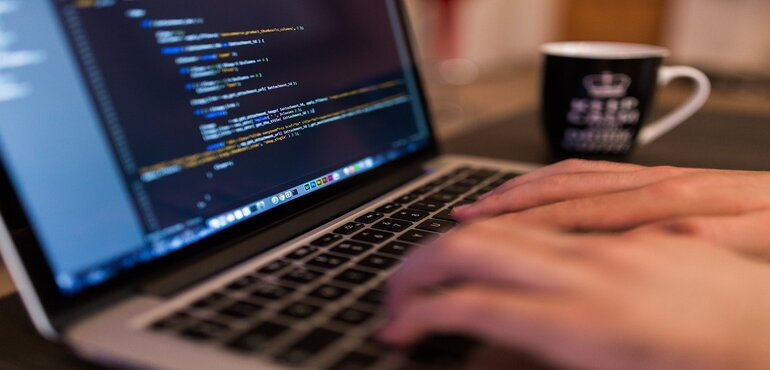Welcome to DigiStackedu10 SEATS

### COURSE INSTRUCTOR### Mahesh Kumar

Sr. Data Scientist

Mr. Mahesh Kumar has 10+ Years of Experience in Data Analytics and Python .

### BASIC INFORMATION

• Price : Call Now!
• Lessons : 14
• Length : 1 Month
• Category : Programming
• Started : 15 Apr 2023
• Shift : 02
• Class : 90

### Course Description

Python Programming is making a revolution in the current IT world and it is used by millions of professionals for data analysis and application development apart from this it also supports functional and object-oriented programming patterns moreover, it is easy to learn and can boost your career in different domains. this course is designed to upgrade student's skills in python core programming.

This course is designed by the best python experts and guides you about a practical approach to development moreover, you will get a live instructor who will solve your doubts and give you assignments on regular intervals.

We will start this course with the basics of python programming and further, we will move to python advanced level libraries that are required to do database connectivity like pymysql apart from this, you will learn how to develop a project using the python programming language.

### Module 1: Python programming Overview

1. Introduction to python.
2. Setting python environment.
3. installation of anaconda.
4. Variables,data type and operators in python.
5. Type casting in Python.
6. Taking User input Python.
7. Basic Problem solving skills in python.
8. Solving Mathmetical equations in Python.
1. Introduction to Conditional Statements.
2. Understanding Basics of If and Else.
3. Controlling the flow of program using IF and Else.
4. User Input with If-Else
5. Nesting of If-Else Staements.
6. Practical Demo on If-Else.
7. Assignments on If-Else Lecture.
8. IF and Else with Loop.
9. Nesting of IF and Else Statements.
1. Introduction to Loop in Python programming
2. Basics of for Loop and While Loop.
3. Combining Loop and If-Else Statements.
4. Positive and Negative Loop.
5. Practical on For Loop in Python programming.
6. Practical on Nested For Loop in Python programming.
7. Practical on While Loop in Python programming.
8. Practical on Nested While Loop in Python programming.
9. For and while Loop on R List.
10. For and while Loop on R Tuples.
11. Switch Case Statement in Python Programming.
1. Introduction to List in Python
2. List with Loop.
3. List inside If and Else Statement.
4. List Append and Extend Function.
5. Delete Element from Python List.
6. Update Python List Elements.
7. Count() function on List.
8. In and Not in Operators on List.
9. List in reverse order
10. Shorting Python List.
11. Assignments on Python List.
1. An Introduction to Tuples.
2. Concept of immutability.
3. Count Operation on Tuples.
4. Arithmetic Operators on Tuples.
5. Checking if two tuples are equal.
6. Which Operations are Not Allowed on Tuples.
7. Assignments on Tuples.
8. Handling duplicates using Python Sets.
9. Working on Set Constructor.
1. An Introduction to Dictionaries in Python.
2. Data Structure of a Dictionary.
3. adding and removing key value pairs.
4. updating key value pairs inside a dictionary
5. Creating dynamic Dictioanries.
6. Dynamic Dictionary Using functions.
7. assignments on Python Dictionaries.
1. An Introduction to Functions in Python.
2. Creating a First Function.
3. Function with parameters.
4. Functions with Return type
5. Function with Python List and Tuples.
6. Functions with Python Loop.
7. Functions for Python Dictionaries.
8. Python lambda Function.
9. Assignment on Python Function.
1. An Introduction to Class in Python.
2. An Introduction to Objects in Python.
3. Creating First Class and Object.
4. Constructors in Python
5. Self Keyword in Python Object Oriented Programming.
6. Inheritance in Python.
8. Assignment on Python Class and Object.
1. An Introduction to File I/O in Python.
3. Writing into a file.
4. Reading a doc file in python
6. Opening and Closing Files.
7. File I/O Modes in Python.
8. Assignment on Python I/O.
1. An Introduction to MySQL.
2. Installing MySQL on Local Machine.
3. Creating Database and tables.
4. Making Connections with MySQL.
5. Inserting records into tables using Python.
6. Updating records of a tables using Python.
7. Deleting records of a tables using Python.
8. Fetching records from tables using Python.
9. Assignment on Python database connections.
1. An Introduction GUI Programming.
2. Designing Forms and Inputs.
3. Creating Buttons in Python.
4. Designing Layouts in Python.
5. Installing GUI Libraries Tkinter.
6. Working on Frame and Menus.
7. Python GUI with MySQL.
8. Assignment on Python GUI.
1. Introduction to Numpy.
2. Working on NumPy Array.
3. Arithmetic Operators on Numpy Array.
4. Inspecting NumPy Array.
5. Subsetting, Slicing, Indexing on Numpy.
6. Manipulation on Numpy Array.
7. Stacking and Splitting Numpy Array.
8. Aggregate Functions on Numpy Array.
9. Copying and Sorting Numpy Array.
1. Introduction to Pandas
2. Creating DataFrames using Pandas
3. Reshaping Data – Changing the layout of a data set
4. Handling Missing values using Pandas
5. Combining Data Sets using Pandas
6. Grouping Data uisng Pandas and Pivot Table.
7. Applying functions on Pandas Data Frame
8. Plotting Time Series using Pandas
1. An Introduction to Project Development.
2. Project Assignments.
3. Report Generation.
4. Final Project Submission.

## Student Reviews

Our Student reviews after completion of this course.